# Formulas

Advanced Product Fields for WooCommerce allows you to create more complex pricing structures through formulas. A formula is a mathematical equation that results in a number.

## Dynamic codes & functions

Aside from numbers and elementary arithmetic operations (+, -, * and /) that make up a basic formula, you can also use dynamic codes. These codes dynamically fill in the number they represent. See a list of all possibilities:

• `[price]` to get the base product price.
• `[qty]` to get the current product quantity.
• `[x]` to get the current field value.
• `[field.{id}]` to get another field value. Replace {id} with your field’s ID as found in the backend. Here’s an example: [field.5e8c41711d1db]
• `[var_yourvarname]` points to a variable. What variables are and how to use them is explained further down.

You can also use some functions in your formulas:

• `min(x;y)` to get the minimum of two options.
• `max(x;y)` to get the maximum of two options.

## Example

Let’s say you’re selling custom wooden shelves. Shelves can be cut in any size the user needs and the price increases as the chosen size increases. An example product page can look like this: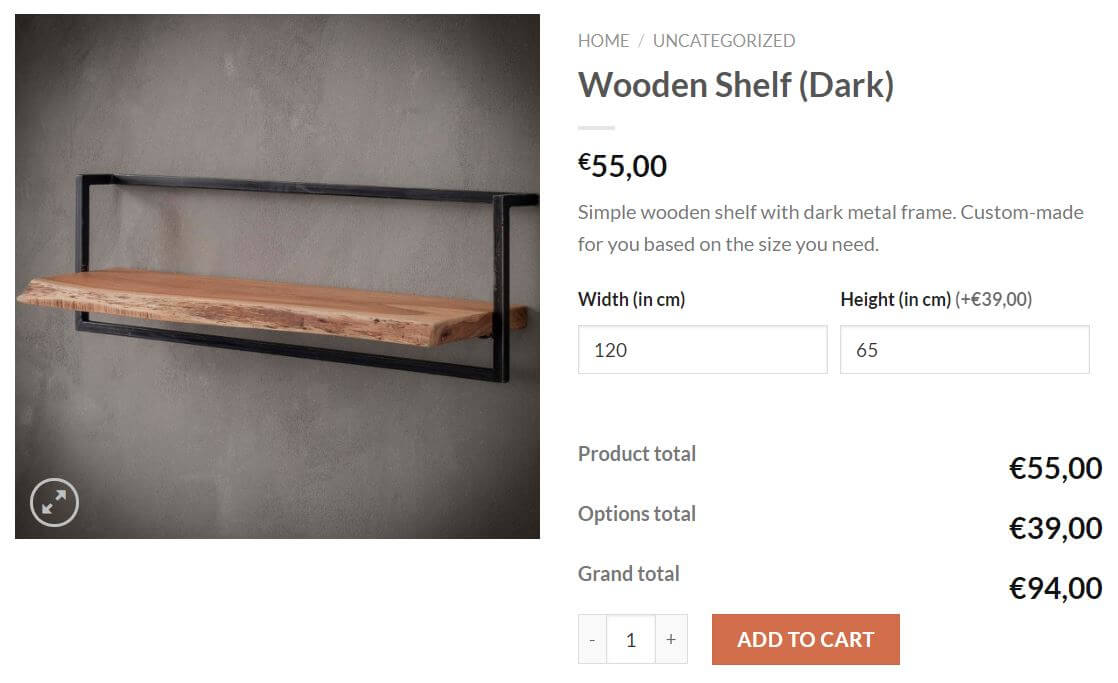The base product costs €55. The additional price depends on the width and height filled out by the user. The formula then calculates the total amount based on a unit price. 1 square mm costs 0.5 cents. The backend looks like this: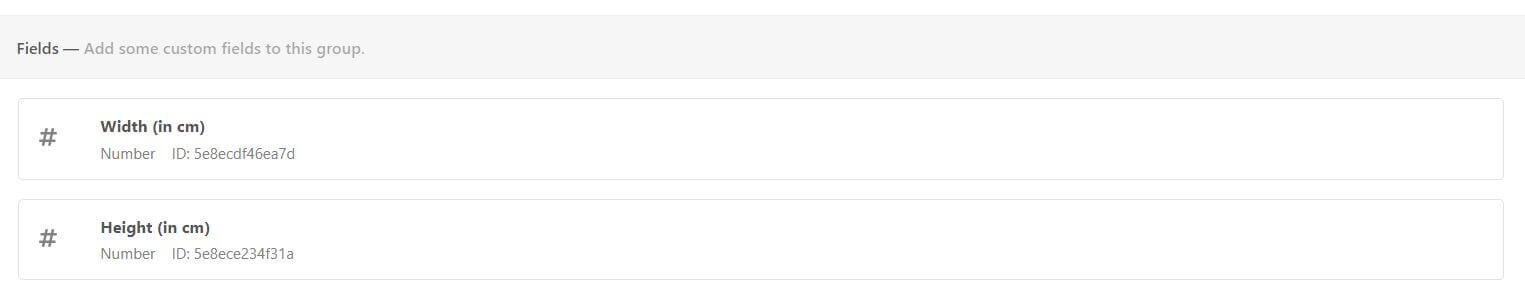We’ve created two number fields. The “height” field has the “pricing” option enabled. The formula used to calculate the price is:

`([field.5e8ecdf46ea7d]*[x])*0.005`

A few things are notable here:

• `[field.5e8ecdf46ea7d]` points to the value of the “width” field.
• `[x]` points to the value of the “height” field itself.
• We multiply this with the unit price for a square centimeter: `0.005` .

# Variables

Advanced Product fields allows you to create variables which can then be used inside formulas. The variable can be static (meaning it only has one value) or dynamic. A dynamic variable can change value depending on other values of product options. Let’s explain it with an example below.

## Example

Let’s say you’re running an online pizza shop. Buyers can choose toppings to put on their pizza at a default price of \$1 per topping. Here’s how this might look on your website: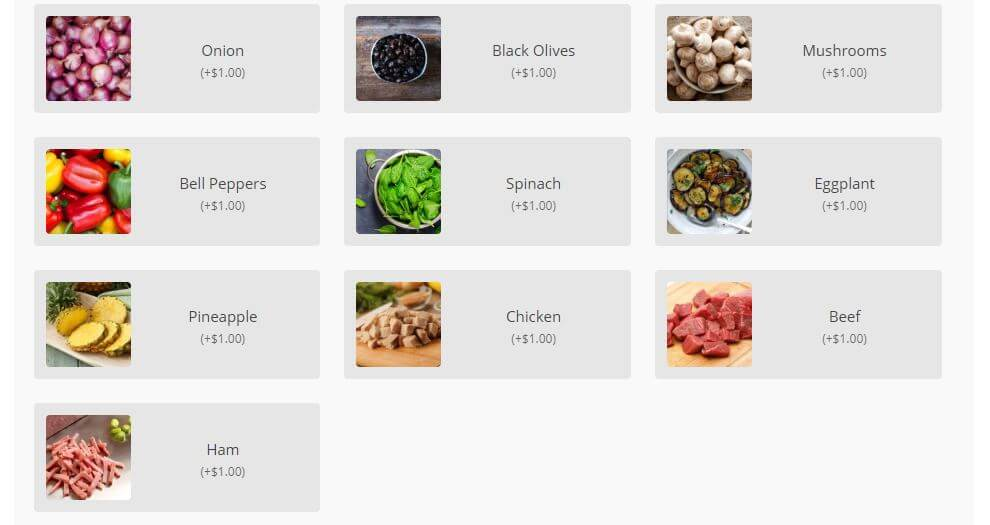Buyers can also choose the size of their pizza. When selecting “Large”, the pizza would be double the size of a small one. So naturally, it would contain more toppings. Therefore, if a user selects “large”, you want each topping to cost \$1.50 instead of the default price of \$1.

This scenario can be solved by creating a variable: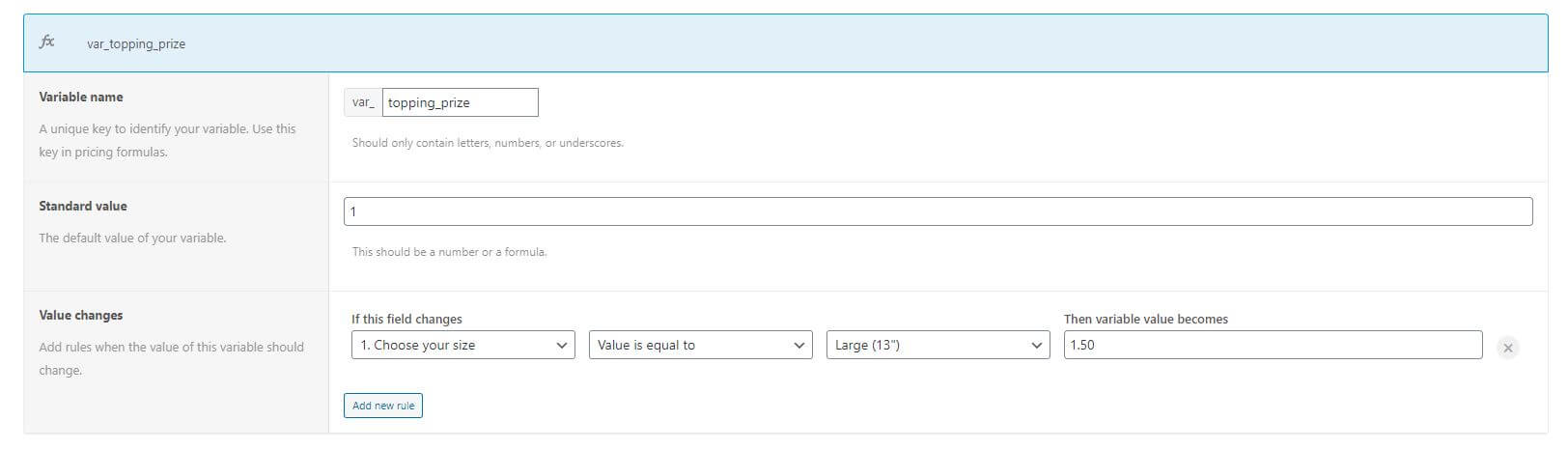First, we gave the variable a name. We called it `topping_prize`. Then, we set the default value to `1` because per default each topping costs \$1.

In the “value changes” section, we add rules to make this variable dynamic. In our case, we need only one rule: when the “Large” size is selected, the variable should be 1.50 instead of 1.

Now, we can use this variable in our topping price settings: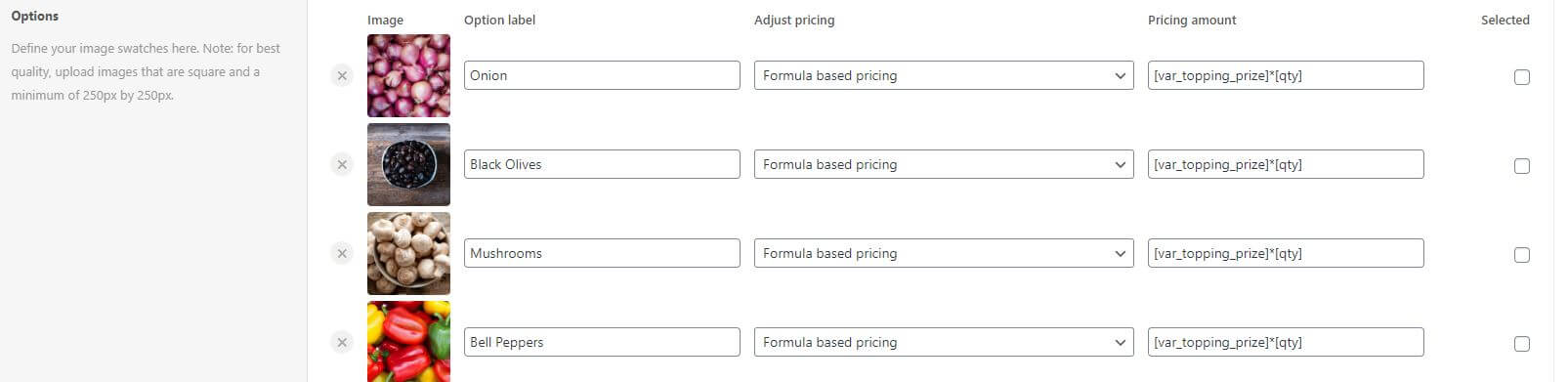The formula is `[var_topping_prize]*[qty]`. Here’s what you should know:

• `[var_topping_prize]` is the name of the variable we created earlier. Note that each variable starts with `var_`.
• We multiply by `[qty]` to make sure that if the user wants two pizzas, the topping prize is also quantity-based.

So now, if the user selects a large pizza, each topping they add will cost \$1.50. In all other cases, toppings cost \$1.